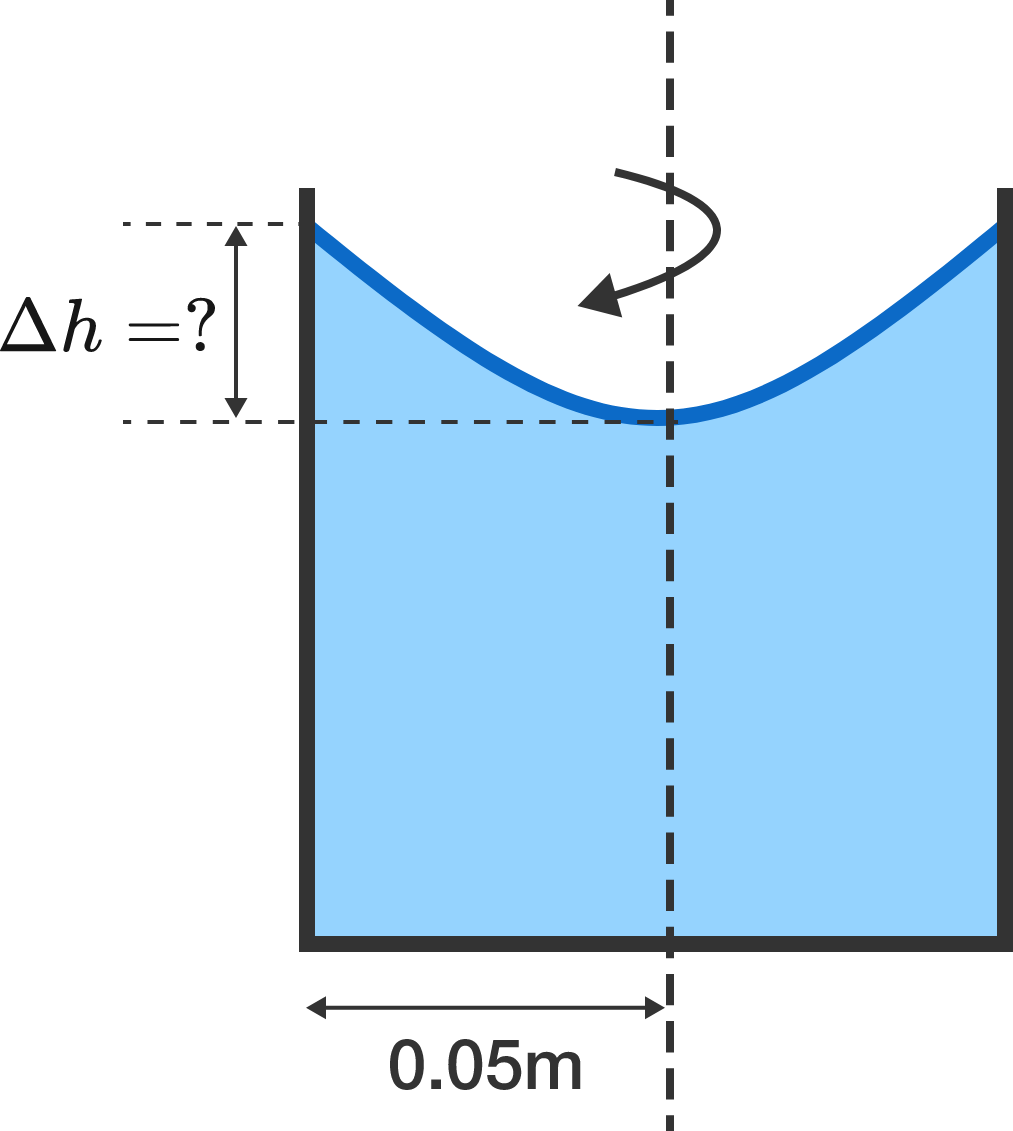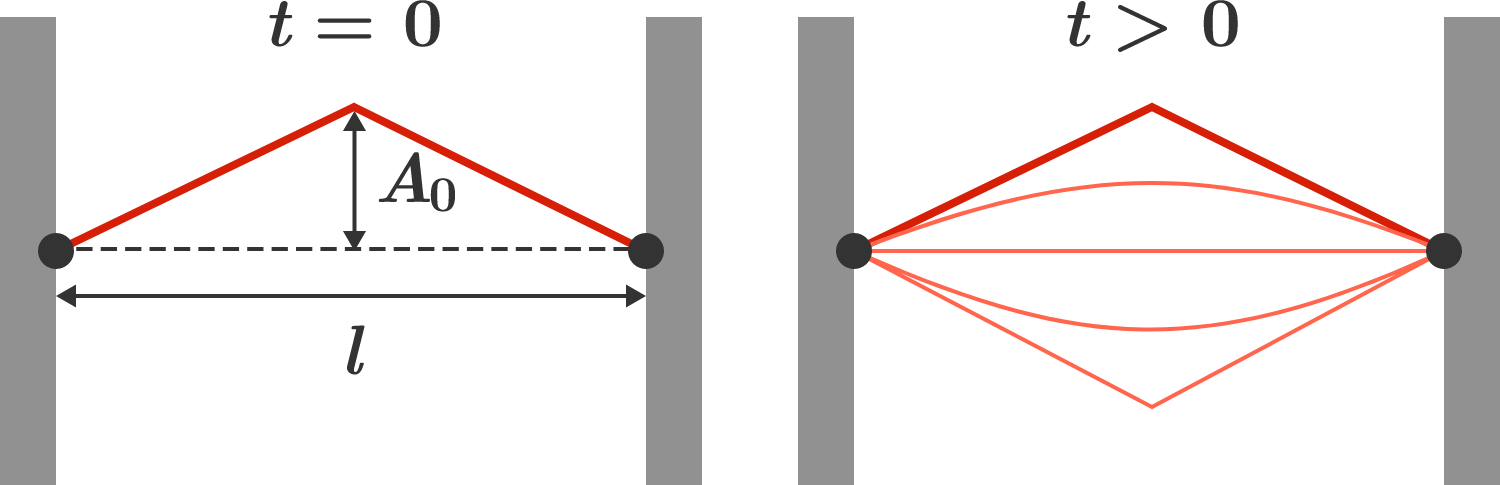# Problems of the Week

Contribute a problem

Consider the equation $x^2+y^2=3z^2.$ Are there any other integer solutions besides the solution where $x=y=z=0?$A liquid is kept in a cylindrical vessel, which is being rotated about its axis. The liquid rises at the side, as shown in the diagram.

If the radius of the vessel is $0.05\text{ m}$ and the speed of rotation is $2$ revolutions per second, find the difference in the heights of the liquid at the center of the vessel and its sides (in centimeters).

Take $g = \pi^2 \text{ m/s}^2.$

Find the number of pairs of positive integers $(a,b)$ with $1\leq a < b \leq 100$ such that

there is at least one positive integer $m$ with $a such that $m$ is divisible by every common divisor of $a$ and $b.$

$\large { \sqrt{20x +\sqrt{20x + \sqrt{20x + 17}}} = 17 }$

Let $N$ be the sum of all the real solutions to the above equation. If $N = \dfrac{a^b-a}{c},$ where $a,$ $b,$ and $c$ are positive integers and $a$ and $c$ are coprime, then what is $a+b+c?$A guitar string of length $l$ stretched along the $x$-axis is plucked in the middle. The initial deflection of the string has the shape $u(x, t = 0) = u_0(x) = \begin{cases} \frac{2 A_0 x}l & x < \frac l2 \\\\ \frac{2 A_0 (l - x)}l & x \geq \frac l2, \end{cases}$ where $A_0$ is in the middle (see diagram). The string is then released and swings freely for time $t > 0,$ and the resulting string vibration can be written as a superposition of standing waves $u(x, t) = \sum_{n = 1}^\infty A_n \cos(2 \pi f_n t) \sin\left( \frac{n\pi}{l} x \right),$ with eigenfrequencies $f_n = n \cdot \nu$ and fundamental frequency $\nu$.

What is the relative amount of vibrational energy stored in the fundamental mode $f = \nu?$ To do this, you'll have to determine the amplitudes $A_n$.

Hint:

• The set of sine functions has the property $\int_0^l \sin\left( \frac{n\pi}{l} x \right) \sin\left( \frac{m\pi}{l} x \right) dx = \begin{cases}\frac l2 & n = m \\ 0 & n \not= m, \quad n, m \in \mathbb{N}. \end{cases}$
• The kinetic energy of a small section of the string at position $x$ of length $dx$ is given by $\frac12 \overbrace{\left(\frac{m}{l}dx\right)}^\textrm{mass} \left(\frac{du(x,t)}{dt}\right)^2,$ where $m$ is the mass of the string. Assume that this holds along the entire string.
×